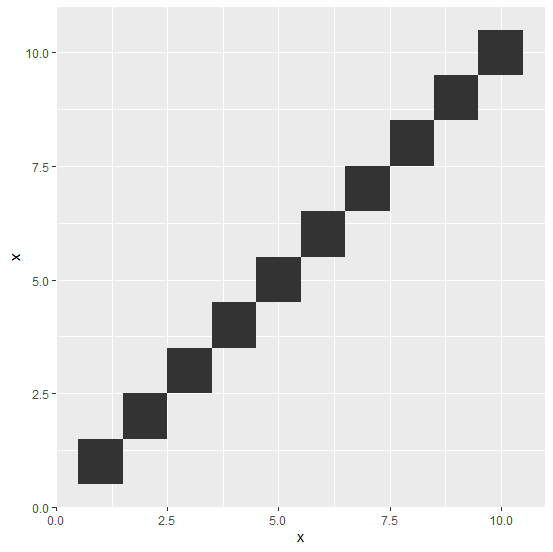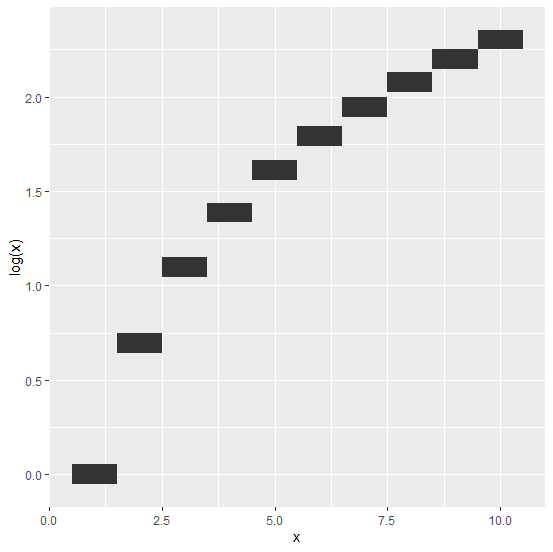# How to create a staircase plot in R?

The simple staircase plot can be created by using geom_tile function of ggplot2 package. We just need to use the vector or the column for which we want to create the staircase plot in place of x and y as well. For example, if we have a column say x of an R data frame df then the staircase plot can be created as ggplot(df,aes(x,x))+geom_tile().

## Example

Consider the below data frame:

Live Demo

> x<-1:10
> df<-data.frame(x)
> df

## Output

x
1 1
2 2
3 3
4 4
5 5
6 6
7 7
8 8
9 9
10 10

> library(ggplot2)
> ggplot(df,aes(x,x))+geom_tile()

## OutputCreating a staircase plot using log function of x:

> ggplot(df,aes(x,log(x)))+geom_tile()

## Output